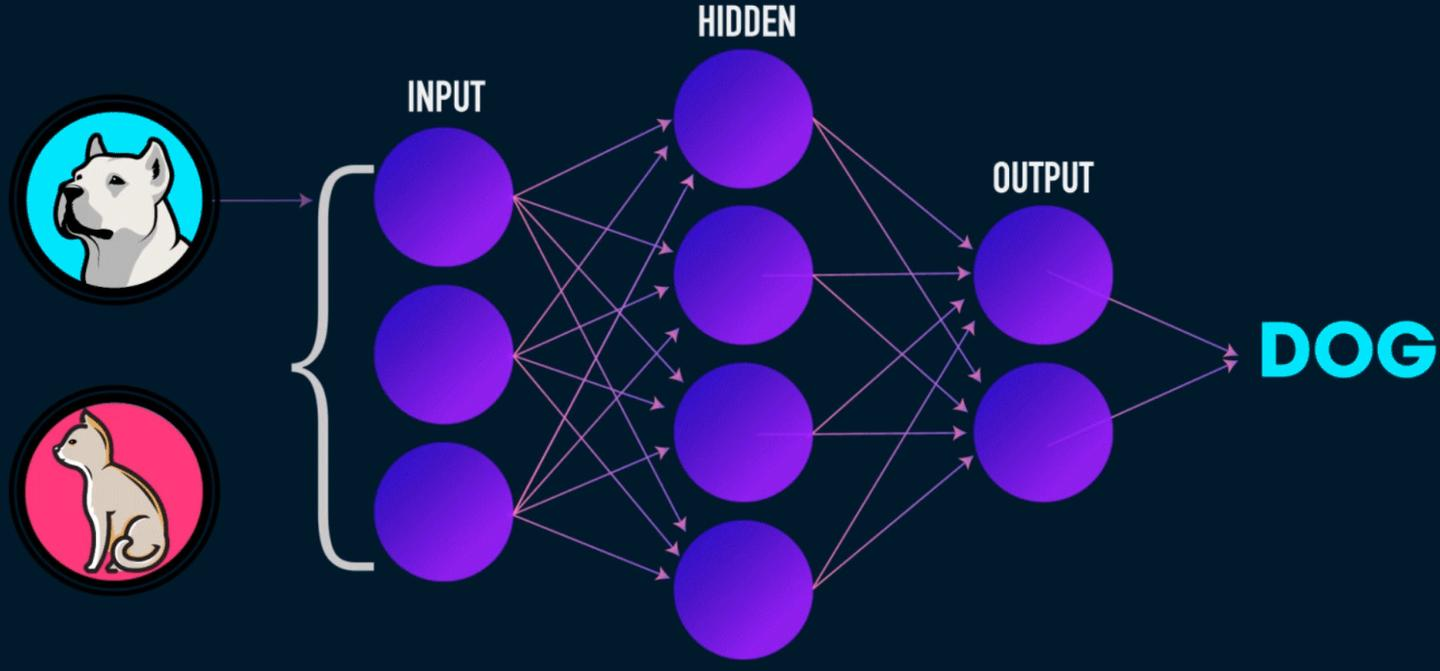• 一个输入层，x
• 任意数量的隐藏层
• 一个输出层，ŷ
• 每两层之间都有一组权重和偏置，W 和 b
• 每个隐藏层都要选择一个激活函数 σ。在本文中，我们选用 Sigmoid 激活函数。

class NeuralNetwork:
def __init__(self, x, y):
self.input      = x
self.weights1   = np.random.rand(self.input.shape,4)
self.weights2   = np.random.rand(4,1)
self.y          = y
self.output     = np.zeros(y.shape)

• 计算预测的输出 ŷ，称为前向传播
• 更新权重和偏置，称为反向传播

class NeuralNetwork:
def __init__(self, x, y):
self.input      = x
self.weights1   = np.random.rand(self.input.shape,4)
self.weights2   = np.random.rand(4,1)
self.y          = y
self.output     = np.zeros(self.y.shape)

def feedforward(self):
self.layer1 = sigmoid(np.dot(self.input, self.weights1))
self.output = sigmoid(np.dot(self.layer1, self.weights2))

class NeuralNetwork:
def __init__(self, x, y):
self.input      = x
self.weights1   = np.random.rand(self.input.shape,4)
self.weights2   = np.random.rand(4,1)
self.y          = y
self.output     = np.zeros(self.y.shape)

def feedforward(self):
self.layer1 = sigmoid(np.dot(self.input, self.weights1))
self.output = sigmoid(np.dot(self.layer1, self.weights2))

def backprop(self):
# application of the chain rule to find derivative of the loss function with respect to weights2 and weights1
d_weights2 = np.dot(self.layer1.T, (2*(self.y - self.output) * sigmoid_derivative(self.output)))
d_weights1 = np.dot(self.input.T,  (np.dot(2*(self.y - self.output) * sigmoid_derivative(self.output), self.weights2.T) * sigmoid_derivative(self.layer1)))

# update the weights with the derivative (slope) of the loss function
self.weights1 += d_weights1
self.weights2 += d_weights2

• 除了 Sigmoid 函数之外，我们还可以使用哪些激活函数？
• 在训练神经网络时使用学习率
• 使用卷积进行图像分类任务